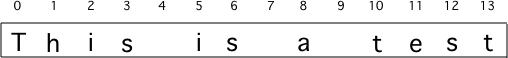# Strings are Objects¶

Strings are objects in Python which means that there is a set of built-in functions that you can use to manipulate strings. You use dot-notation to invoke the functions on a string object such as `sentence.lower()`. The function `lower()` returns a new string with all of the characters in the original string set to lowercase. The function `capitalize()` will return a new string with the first letter of the string capitalized.

## Getting Part of a String¶

A string has characters in a sequence. Each character is at a position or index which starts with 0 as shown below. An index is a number associated with a position in a collection of values like a string.Figure 1: A string with the position (index) shown above each character

A slice is a way to get part of a string. One way to use a slice is to do stringName[num]`. This will return a new string with just the character at that position in the string.Figure 2: A string with the position (index) shown above each character

A slice with two values separated with a `:` between them returns a new string with the characters from the given start position to the one before the given end position.

csp-4-2-1: What will be printed when the following executes?

```str = "This is the end"
str = str[1:4]
print(str)
```
• This is the end
• This would be true if we were printing the value of str and we hand't changed it on line 2.
• This
• This would be true if the first position was 1 and the substring included the character at the end position, but the first character in a string is at position 0 and the substring won't include the character at the last position.
• his
• This will return a string that starts at position 1 and ends at position 3.

csp-4-2-2: What will be printed when the following executes?

```str = "This is the end"
str = str
print(str)
```
• i
• This will print the character at position 5 in the string which is i. Remember that the first character is at position 0.
• s
• This would be true if the first character was at position 1 instead of 0.
• is the end
• This would be true if it returned from the given position to the end of the string, but that isn't what it does.

## Some Other String Functions¶

The `len(string)` function takes a string as input and returns the number of characters in the string including spaces.

The `find(string)` function takes a string as input and returns the index where that string is found in the current string. If the string isn’t found it returns -1.

Note

The `find` function will return the first position it finds the given string in. Notice that above it printed 2 which means it found the “is” in “This” first.

csp-4-2-3: Given the following code segment, what will be printed?

```street = "125 Main Street"
print(len(street))
```
• 13
• Don't forget to include the spaces in the count.
• 15
• The len function returns the number of elements in the string including spaces.
• 10
• This would be true if the len function only returned the number of alphabetic characters, but it includes all including spaces.

csp-4-2-4: What will be printed when the following executes?

```str = "His shirt is red"
pos = str.find("is")
print(pos)
```
• 1
• The find function returns the index of the first position that contains the given string.
• 9
• This would be true if it was pos = str.find(" is").
• 10
• This would be true if it was pos = str.find(" is") and the first position was 1, but it is 0.

Write the code to evaluate the length of the string “I like green eggs” and print it. It should print “The length is 17”.

Create variable to hold the string.